## Adding the Spins of Two Electrons

The coordinates of two particles commute with each other:. They are independent variables except that the overall wave functions for identical particles must satisfy the (anti)symmetrization requirements. This will also be the case for the spin coordinates.We define the total spin operatorsIts easy to show the total spin operators obey the same commutation relations as individual spin operatorsThis is a very important result since we derived everything about angular momentum from the commutators. The sum of angular momentum will be quantized in the same way as orbital angular momentum.

As with the combination of independent spatial coordinates, we can make product statesto describe the spins of two particles. These products just mean, for example, the spin of particle 1 is up and the spin of particle 2 is down. There are four possible (product) spin states when we combine two spinparticles. These product states are eigenstates of totalbut not necessarily of total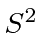. The states and their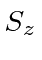eigenvalues are given below.

 Product State Totaleigenvalue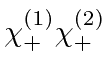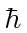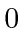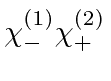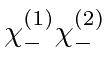Verify the quoted eigenvalues by calculation using the operator.

We expect to be able to form eigenstates offrom linear combinations of these four states. From pure counting of the number of states for eacheigenvalue, we can guess that we can make onemultiplet plus onemultiplet. Themultiplet has three component states, two of which are obvious from the list above. We can use the lowering operator to derive the other eigenstates of.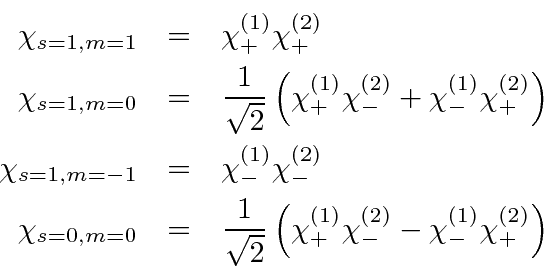As a necessary check, we operate on these states withand verify that they are indeed the correct eigenstates.

Note that by deciding to add the spins together, we could not change the nature of the electrons. They are still spinand hence, these are all still eigenstates ofand, however, (some of) the above states are not eigenstates ofand. This will prove to be a general feature of adding angular momenta. Our states of definite total angular momentum and z component of total angular momentum will still also be eigenstates of the individual angular momenta squared.

Jim Branson 2013-04-22